CBSE Class 12 Sample Paper for 2018 Boards

Class 12
Solutions of Sample Papers and Past Year Papers - for Class 12 Boards

### Four bad oranges are accidentally mixed with 16 good ones. Find the probability distribution of the number of bad oranges when two oranges are drawn at random from this lot. Find the mean and variance of the distribution.

This is a question of CBSE Sample Paper - Class 12 - 2017/18.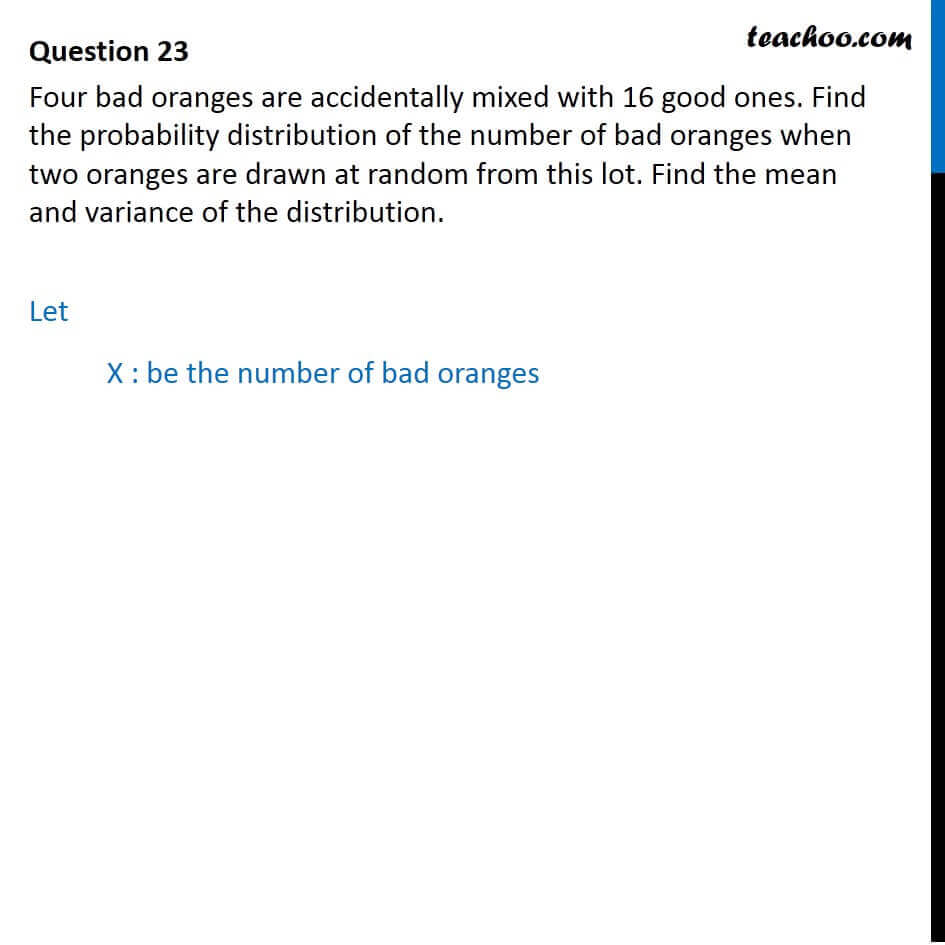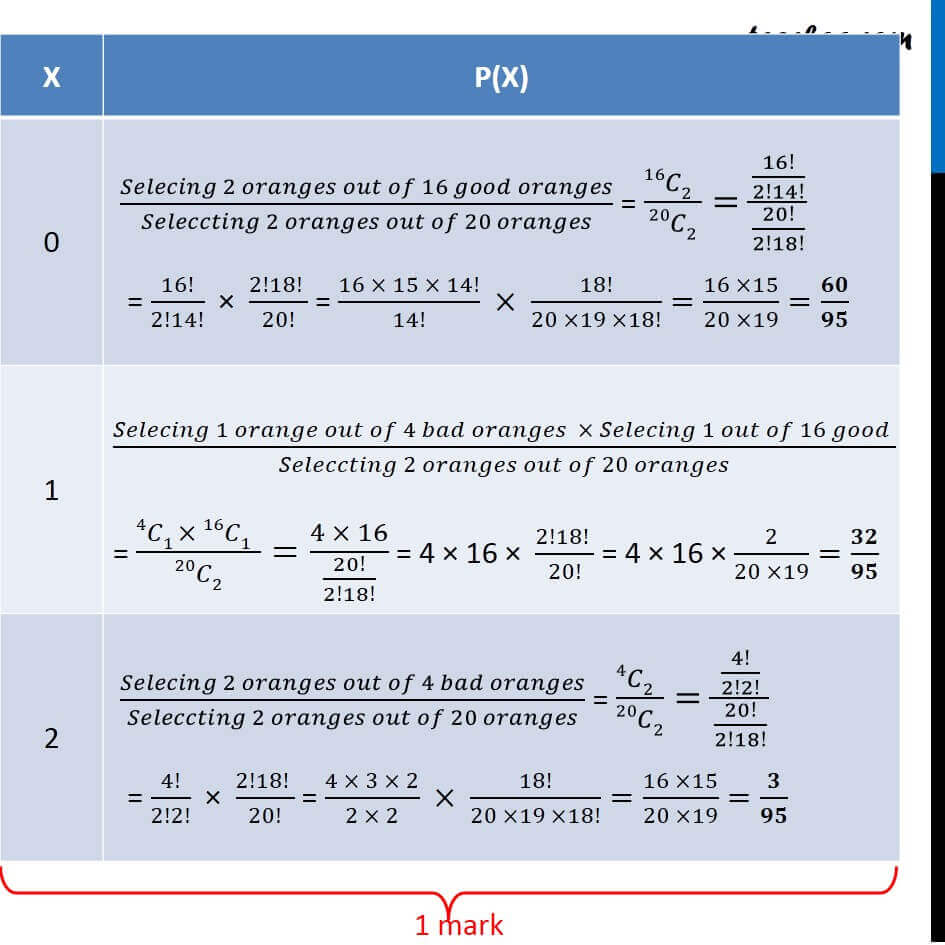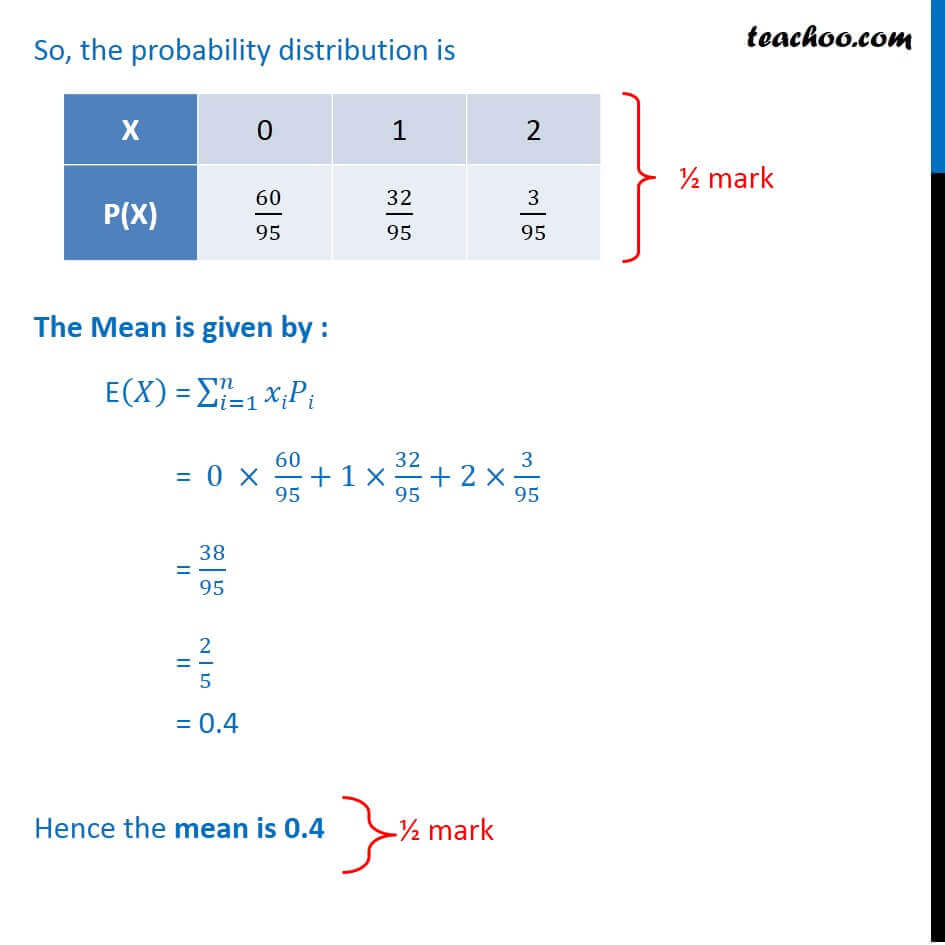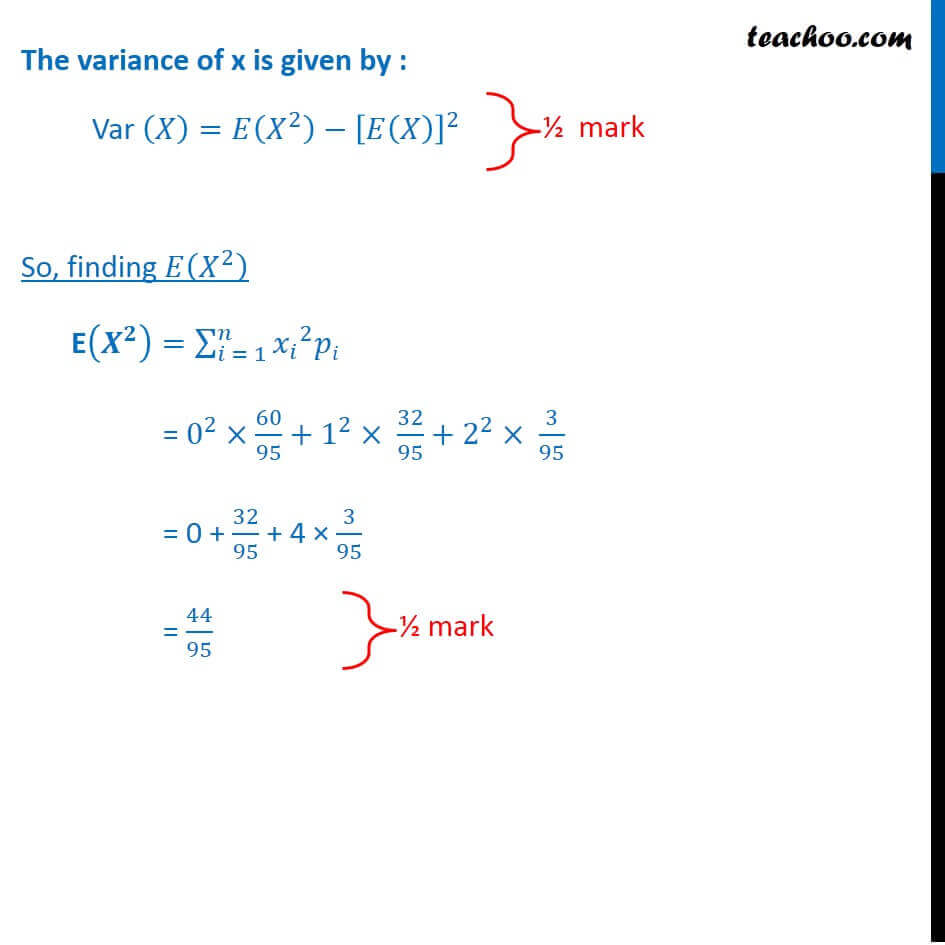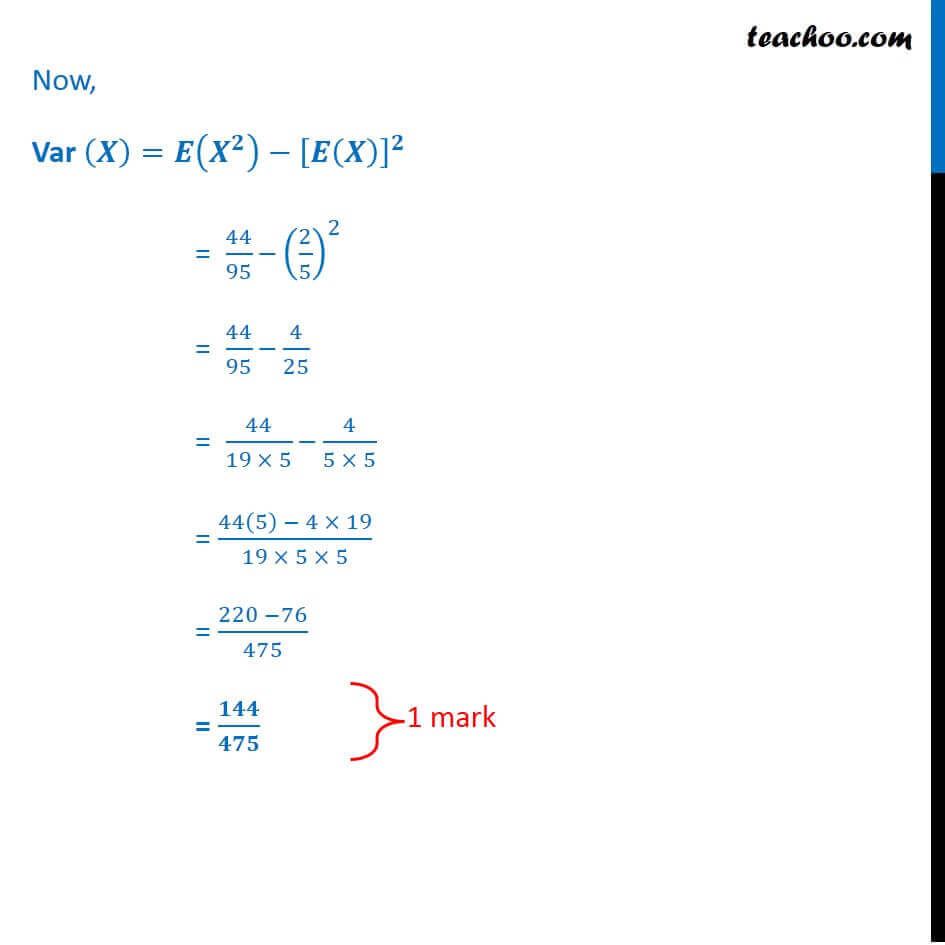Learn in your speed, with individual attention - Teachoo Maths 1-on-1 Class

### Transcript

Let X : be the number of bad oranges x 0 1 2 The Mean is given by : E( )" =" 2_( =1)^ = 0 60/95+1 32/95+2 3/95 = 38/95 = 2/5 = 0.4 Hence the mean is 0.4 The variance of x is given by : Var ( )= ( ^2 ) [ ( )]^2 So, finding ( ^2 ) E( ^ )= 2_( = 1)^ _ ^2 = 0^2 60/95+1^2 32/95+2^2 3/95 = 0 + 32/95 + 4 3/95 = 44/95 Now, Var ( )= ( ^ ) [ ( )]^ = 44/95 (2/5)^2 = 44/95 4/25 = 44/(19 5) 4/(5 5) = (44(5) 4 19)/(19 5 5) = (220 76)/475 = /